### DEPLAG Statement

DEPLAG LAGS = order <PHI = value …> <NOEST> ;

The DEPLAG statement is used to specify the lags of the dependent variable to be included as predictors in the model. The following examples illustrate the use of DEPLAG statement.

If the dependent series is denoted by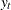, the following statement specifies the inclusion of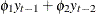in the model. The parameters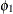and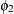are estimated from the data.

      deplag lags=2;


The following statement requests including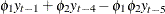in the model. The values ofandare fixed at 0.8 and –1.2.

      deplag lags=(1)(4) phi=0.8 -1.2 noest;


The dependent lag parameters are not constrained to lie in any particular region. In particular, this implies that a UCM that contains only an irregular component and dependent lags, resulting in a traditional autoregressive model, is not constrained to be a stationary model. In the DEPLAG statement, if an initial value is supplied for any one of the parameters, the initial values must also be supplied for all other parameters.

LAGS=order
LAGS=(lag, …, lag ) …(lag, …, lag )

is a required option in this statement. LAGS=(l, l, …, l) defines a model with specified lags of the dependent variable included as predictors. LAGS=order is equivalent to LAGS=(1, 2, …, order ).

A concatenation of parenthesized lists specifies a factored model. For example, LAGS=(1)(12) specifies that the lag values, 1, 12, and 13, corresponding to the following polynomial in the backward shift operator, be included in the model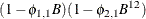Note that, in this case, the coefficient of the thirteenth lag is constrained to be the product of the coefficients of the first and twelfth lags.

NOEST

fixes the values of the parameters to those specified in PHI= option.

PHI=value

lists starting values for the coefficients of the lagged dependent variable. The order of the values listed corresponds with the order of the lags specified in the LAGS= option.